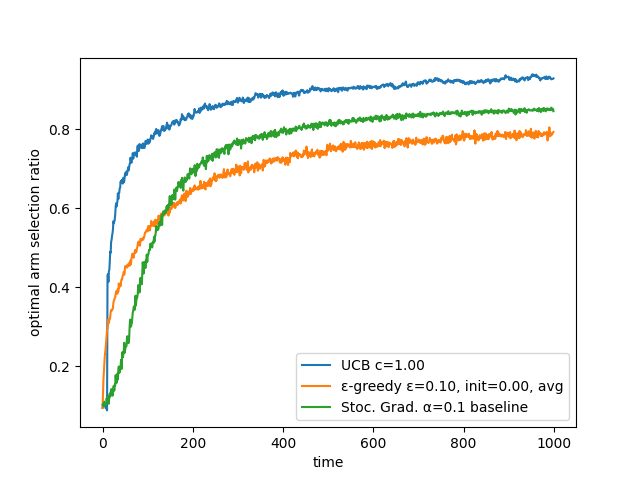## rl-bandit

A simple multi-armed bandit implementation

### 3 stable releases

 1.0.2 Sep 14, 2020

#201 in Algorithms

49KB
195 lines

# rl-bandit: A rust bandit implementation

Simple multi-armed bandit algorithm implementation.

Implements several bandit algorithms (most of them found in ``Reinforcement Learning: An Introduction'' by Richard S. Sutton and Andrew G. Barto. It is available for free at http://www.incompleteideas.net/book/the-book-2nd.html).# Usage example

Initialize the bandit algorithm (a few examples)

``````// ε-greedy algorithm with 10 arms, ε=0.1, initial values of 0
let egreedy1 = EGreedy::new(10, 0.1, 0.0, UpdateType::Average));

// same ε-greedy but non-stationary with step size of 0.1
let egreedy2 = EGreedy::new(10, 0.1, 0.0, UpdateType::Nonstationary(0.1));

// Upper Confidence Bound with 10 arms and c=1
let ucb1 = UCB::new(10, 1.);

// Stochastic gradient with 10 arms, step size of 0.1, with baseline
let sg1 = StochasticGradient::new(10, 0.1, true);
``````

feedback loop:

``````// choose the best action according to the bandit algorithm
let action = ucb1.choose();

let reward = [...]; // using the action and computing the reward

// updates the bandit algorithm using the reward
ucb1.update(action, reward);
``````

Note: A more detailed example and benchmark can be found in the rl-bandit-bench crate.

# implemented algorithms:

• ε-greedy
• optimistic ε-greedy
• Upper-Confidence-Bound (UCB)